# Algebra II : Multiplication and Division

## Example Questions

1 2 3 4 5 6 7 9 Next →

### Example Question #81 : Multiplication And Division

Multiply the numbers: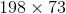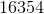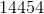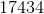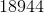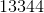Explanation:

Multiply the first number given with the ones digit of 73.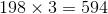Multiply the first number given with the tens digit of 73.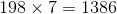Add an extra zero to the end of this number and add this with the first number.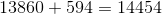The answer is:### Example Question #2386 : Mathematical Relationships And Basic Graphs

Try without a calculator:

Which is true about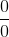?is an undefined quantity.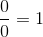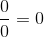None of these

All of theseis an undefined quantity.